# Mushroom cultivation

Control the parameters of temperature, humidity, lighting and check the access to the crop to automate the process.

EasyShowcase (no instructions)8 hours1,088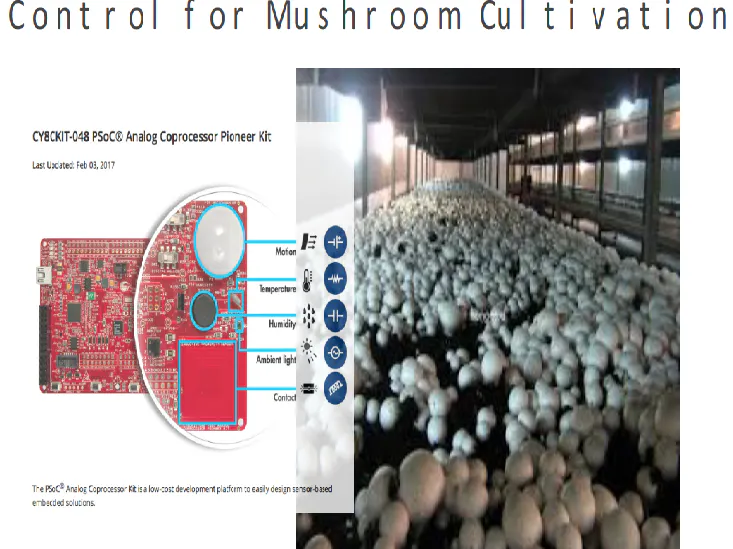## Things used in this project

### Hardware components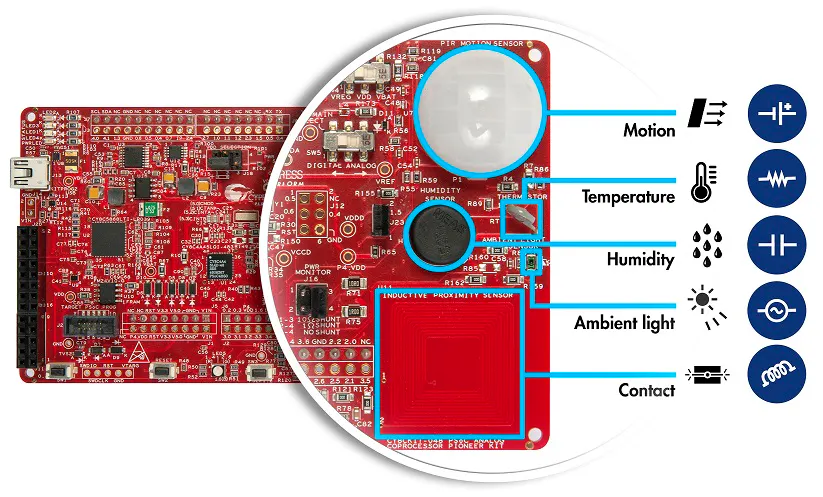Cypress PSoC Analog Coprocessor Pioneer Kit
×1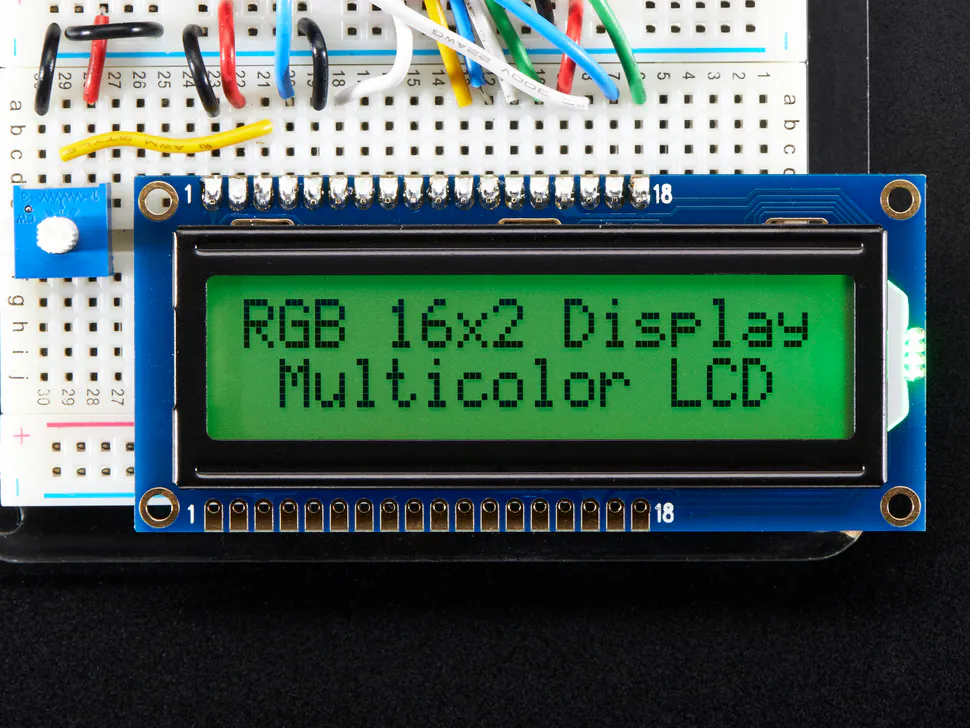Adafruit RGB Backlight LCD - 16x2
×1LED (generic)
×4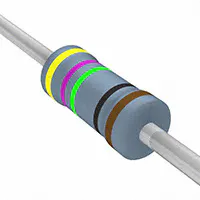Resistor 475 ohm
×4

### Software apps and online services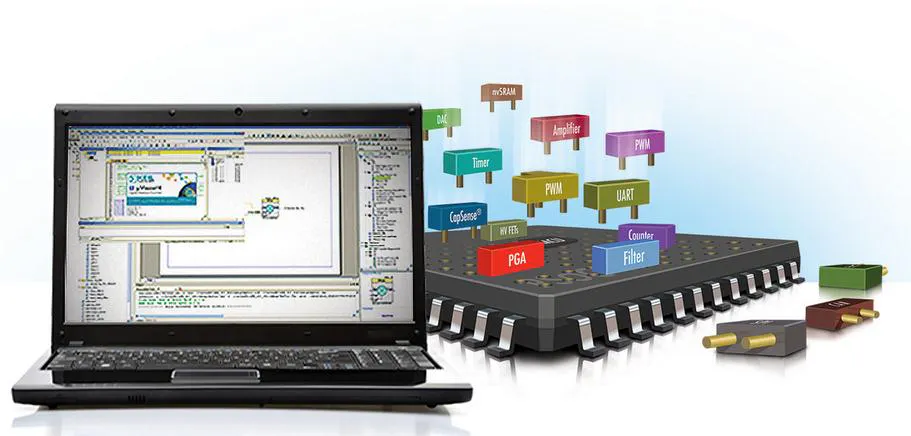Cypress PSoC Creator

## Schematics

### Schematic## Code

### Code

C/C++
```/* ========================================
*
* UNPUBLISHED, LICENSED SOFTWARE.
*
* CONFIDENTIAL AND PROPRIETARY INFORMATION
* WHICH IS THE PROPERTY OF your company.
* Credits: Ing. Jorge Eduardo Porras B.
* ========================================
*/
#include "project.h"
#include <stdio.h>
#include "LiquidCrystal_I2C.h"

/* LCD Address */

int16 temp, hume;
char mensaje;

//****TEMPERATURE SENSOR VARIABLES

// /* Variables to hold the the ADC readings */

///* Filter input and output variables for Vref and Vth measurements */
int16 filterOutputVref=0;
int16 filterOutputVth=0;
//
///* Variables to hold calculated resistance and temperature */
int16 thermistorResistance, temperature;

#define FILTER_COEFFICIENT_TEMPERATURE	(32u)

//****MOISTURE SENSOR VARIABLES

uint16 humidityRawCounts;	/* Raw count from CapSense Component for the humidity sensor */
uint16 capacitance;			/* Capacitance of the humidity sensor */
uint16 humidity;			/* Measured humidity */
uint16 rawCountsRefCap;     /* Raw count from CapSense Component for the Reference capacitor */

#define CAPACITANCE_AT_55_RH        (1800)
/* Sensitivity numerator and denominator indicate sensitivity of the sensor */
#define SENSITIVITY_NUMERATOR       (31)
#define SENSITIVITY_DENOMINATOR     (100)
/* Value of reference capacitor.  Note that this value includes the pin capacitance
and the physical 180pF reference capacitor */
#define CREF                        (1930)
/* Offset Capacitance */
#define COFFSET                     (150)
/* This is raw count equivalent to trace capacitance */
#define OFFSETCOUNT                 (1536)
#define BUFFERSIZE                  (8)
/* Nominal humidity 55% */
#define NOMINAL_HUMIDITY            (550)
#define HUMIDITY_0_PERCENT          (0)
#define HUMIDITY_100_PERCENT        (1000)
#define HUMIDITY_50                 (500)

//****LIGHTING SENSOR VARIABLES

/* This variable is used to store the ADC result */

/* These are used for firmware low pass filter input and output */
int16 filterInput;
int32 filterOutput = 0;

/* Variable to store sensor current and light illuminance */
int16 alsCurrent;
uint16 illuminance;
int16 alsCurrent;			/* Ambient light sensor current output */
uint16 illuminance; 		/* Ambient light illuminance */

#define FILTER_COEFFICIENT_ALS			        (10)

/* Constants for photodiode current calculation */
/* Scale Factor = (VREF / (2048 * 220K)) * 10^9 nA = 2.6633
As the TIA produces a negative voltage, the scale factor is made
negative */
#define ALS_CURRENT_SCALE_FACTOR_NUMERATOR		(-26633)
#define ALS_CURRENT_SCALE_FACTOR_DENOMINATOR	(10000)

/* Constants for ambient light calculation */
/* Scale Factor = 10000Lx / 3000nA = 3.333 */
#define ALS_LIGHT_SCALE_FACTOR_NUMERATOR		(3333)
#define ALS_LIGHT_SCALE_FACTOR_DENOMINATOR		(1000)

#define PWM_DUTY_SCALE	         (1u)
#define PWM_DUTY_OFFSET	         (0)

__inline uint16 CalculateCapacitance(uint16 rawCounts, uint16 refSensorCounts);
__inline uint16 CalculateHumidity(uint16 capacitance);

int main(void)
{
CyGlobalIntEnable; /* Enable global interrupts. */

/* Place your initialization/startup code here (e.g. MyInst_Start()) */
I2C_Start();
CSD_Start();
/* Start Reference buffer */
VrefBuffer_Start();
/* Start Programmable Voltage Reference */
PVref_Start();
/* Enable Programmable Voltage Reference */
PVref_Enable();
/* Start the trans-impedance amplifier (TIA) */
Opamp_TIA_Start();
/* Start Reference buffer */
RefBuffer_Start();
/* Start Programmable Voltage Reference */
PVref_1_Start();
/* Enable Programmable Voltage Reference */
PVref_1_Enable();

begin();

for(;;)
{
/* Place your application code here. */

//TEMPERATURE CALCULATIONS

/* Low pass filter the measured ADC counts of Vref */
filterOutputVref = (adcResultVREF + (FILTER_COEFFICIENT_TEMPERATURE - 1) * filterOutputVref) / FILTER_COEFFICIENT_TEMPERATURE;
/* Low pass filter the measured ADC counts of Vth */
filterOutputVth = (adcResultVTH + (FILTER_COEFFICIENT_TEMPERATURE - 1) * filterOutputVth) / FILTER_COEFFICIENT_TEMPERATURE;
/* Calculate thermistor resistance */
thermistorResistance = Thermistor_GetResistance(filterOutputVref, filterOutputVth);
/* Calculate temperature in degree Celsius using the Component API */
temperature = Thermistor_GetTemperature(thermistorResistance);
temp=temperature*0.01;

//LIGHTING CALCULATIONS

/* Low pass filter the ADC result */
filterOutput = (filterInput + (FILTER_COEFFICIENT_ALS - 1)*filterOutput)/FILTER_COEFFICIENT_ALS;
/* Calculate the photodiode current */
alsCurrent = (filterOutput * ALS_CURRENT_SCALE_FACTOR_NUMERATOR)/ALS_CURRENT_SCALE_FACTOR_DENOMINATOR;
/* If the calculated current is negative, limit it to zero */
if(alsCurrent < 0)
{
alsCurrent = 0;
}
/* Calculate the light illuminance */
illuminance = (alsCurrent * ALS_LIGHT_SCALE_FACTOR_NUMERATOR)/ALS_LIGHT_SCALE_FACTOR_DENOMINATOR;

//MOISTURE CALCULATIONS

if(!(CSD_IsBusy()))
{
humidityRawCounts = CSD_BUTTON0_SNS0_RAW0_VALUE;
rawCountsRefCap = CSD_BUTTON0_SNS1_RAW0_VALUE;
/* Convert raw counts to capacitance */
capacitance = CalculateCapacitance(humidityRawCounts, rawCountsRefCap);
/*Calculate humidity */
humidity = CalculateHumidity(capacitance);
hume=humidity*0.1;
CSD_ScanAllWidgets();
}

sprintf(mensaje,"Te=%.2i   ",temp);
setCursor(0,0);
LCD_print(mensaje);
setCursor(5,0);
LCD_print("C");

sprintf(mensaje,"Lx=%.2i   ",illuminance);
setCursor(0,1);
LCD_print(mensaje);

sprintf(mensaje,"Hu=%.2i   ",hume);
setCursor(8,0);
LCD_print(mensaje);
setCursor(14,0);
LCD_print("%");

//GREENHOUSE CONTROL

if (temp <24) {
Control_Calefactor_Write(1u);
}
if ((temp >=24) & (temp<=26) ) {
Control_Calefactor_Write(0u);
}
if (temp >26) {
Control_Calefactor_Write(0u);
}
if (hume <80) {
}
if (hume >=82) {
}
if (illuminance <100) {
Control_Iluminacion_Write(1u);
}
if (illuminance >=102) {
Control_Iluminacion_Write(0u);
}

CyDelay(250u);//milisegundos

}
}

__inline uint16 CalculateCapacitance(uint16 rawCounts, uint16 refsensorCounts)
{
return (uint16)((float)(rawCounts - OFFSETCOUNT) * (CREF - COFFSET) / (float)(refsensorCounts - OFFSETCOUNT));
}

__inline uint16 CalculateHumidity(uint16 capacitance)
{
int16 humidity;
int16 delta;
/* Find capacitance difference from nominal capacitance at 55% RH */
delta = capacitance - CAPACITANCE_AT_55_RH;
/* Calculate humidity from capacitance difference and sensor sensitivity */
humidity = ((delta * SENSITIVITY_DENOMINATOR) / SENSITIVITY_NUMERATOR) + NOMINAL_HUMIDITY;
/* If humidity is less than zero, limit it to 0; If humidity is greater than 1000 (100%), limit to 1000 */
humidity = (humidity < HUMIDITY_0_PERCENT) ? HUMIDITY_0_PERCENT : (humidity > HUMIDITY_100_PERCENT) ? HUMIDITY_100_PERCENT : humidity;
/* Return Humidity value */
return humidity;
}

/* [] END OF FILE */
```

## Credits

### Jorge Eduardo Porras B

1 project • 2 followers